##Good Parametrizations / Stereographic projections

I borrow the term from Marcel Berger. For every point C of the conic (c) there is a natural bijection of the points of the conic to the set C* of the lines Lc, passing through C. To each point X of the conic we simply associate the line LX=[CX]. To the point C itself corresponds the tangent to (c) at C. Since C* is a line in the dual projective plane, this can be considered as a parametrization of the conic through the points of a line. This is the idea of the good parametrization(++).

From the invariant definition of this parametrization one can construct coordinate systems and parametrizations of the conic, by using the idea of stereographic projection. In fact, take an arbitrary line (e) and map every point X of the conic to the point Y = fC(X), defined by the intersection of (e) with line LX = [CX]. fC is a bijection of the points of (c) (and of C*) onto the points of the (projective) line (e).

I call these also stereographic projections of the conic. Sometimes I use for them also the term good parametrizations. The use of a line (e') different from (e) introduces a linear relation (s'=as+bt, t'=cs+dt) between the projective coordinates (s,t) and (s',t') of the two lines. This is a consequence of the invariance of the cross-ratio along a bundle of lines passing through the same point C.

Define the analogous map fD(X) for another point D of the conic. The "transition" function g(x) = fD(fC-1(x)) is a homographic relation for the coordinate x of the line (e). This is an immediate consequence of the invertibility of g and the fact that it is defined through operations involving intersections of lines with quadratic curves.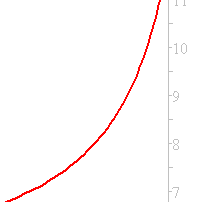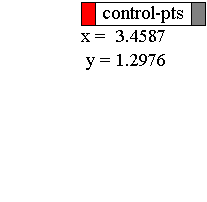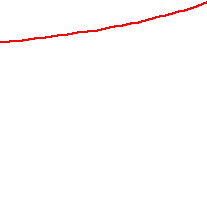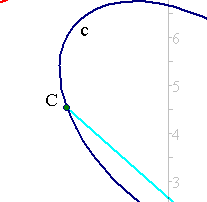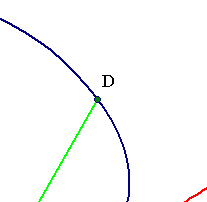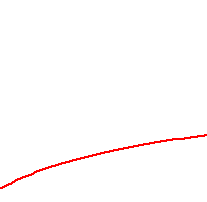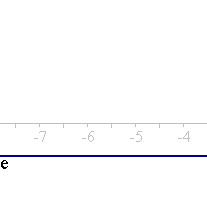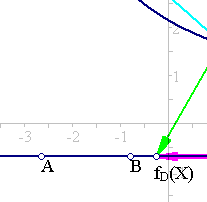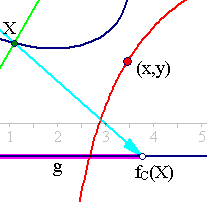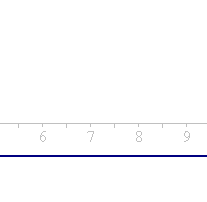The argument can be reversed: Consider two points C, D and a homographic relation y=g(x) on the points of a line (e). The intersection points X of the lines Cx, Dy, joining corresponding points of the line build a conic. This again is obvious, since eliminating the line coordinate from the equation defining X leads to a quadratic equation for the coordinates X(x1, x2) of X. This kind of generation of the conic, through a homographic relation is called a Chasles-Steiner generation of the conic.
Some of the applications of the idea of good parametrization are the definitions of cross ratio of four points on a conic (discussed in the file CrossRatio.html ), and the definitions of homography and its particular case, the involution on a conic, both discussed in the files referred below.
The inverse of a good parametrization is a rational representation of the curve in terms of the (projective) coordinates of a line. Indeed, setting (u,v) for a system of projective coordinates of the line (e) and working out the inverse of fD, we find that X is given in terms of (u,v) through quadratic polynomials (see GoodParametrizationInverse.html for the details):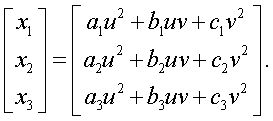Good parametrizations generalize the stereographic projection of the circle. Look at Stereographic.html for the discussion of this particular example.
(++) Good parametrizations establish a bijection (homeomorphism) between projective conics and projective lines, showing that conic sections are bendings of lines. A further common characteristic with the lines is that all (projective) conics are equivalent under projectivities. There are many ways to see that. A simple one is through a particular coordinate system, adapted to an arbitrary conic, in which that conic is represented through the equation x2 = yz. This is discussed in GoodParametrizationInverse.html .

Chasles_Steiner.html
Chasles_Steiner_Envelope.html
Complex_Cross_Ratio.html
Complex_Cross_Ratio2.html
CrossRatio.html
GoodParametrizationInverse.html
Harmonic_Bundle.html
HomographicRelation.html
HomographicRelationExample.html
ParabolaProperty.html
ProjectiveBase.html
ProjectiveCoordinates.html
ProjectivePlane.html
RectHypeRelation.html
Stereographic.html

### References

Berger, M. Geometry I, II Paris, Springer Verlag 1987, vol. II, par. 16.2, p. 173.
Berger Marcel, Pansu Pierre, Berry Jean-Pic, Saint-Raymond Xavier Problems in Geometry Paris, Springer Verlag 1984, p. 94.